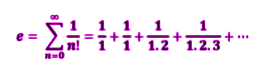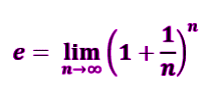Checkout JEE MAINS 2022 Question Paper Analysis : Checkout JEE MAINS 2022 Question Paper Analysis :

# Value of e

Euler’s Number ‘e’ is a numerical constant used in mathematical calculations. The value of e is 2.718281828459045…so on. Just like pi(π), e is also an irrational number. It is described basically under logarithm concepts. ‘e’ is a mathematical constant, which is basically the base of the natural logarithm. This is an important constant which is used in not only Mathematics but also in Physics. It is also called as the Eulerian Number or Napier’s Constant.

‘E’ is majorly used to represent the non-linear increase or decrease of a function such as growth or decay of population. The major application can be seen in exponential distribution.

Value of e to the power 1 (e1) will give the same value as e but the value of e to the power 0 (e0) is equal to 1 and e raised to the power infinity gives the value as 0. It is a unique and special number, whose logarithm gives the value as 1, i.e.,

Log e = 1

In this article, we will learn to evaluate the value of Euler’s number.

## Euler’s Number (e)

The Euler’s number ‘e’, is the limit of (1 + 1/n)n as n approaches infinity, an expression that arises in the study of compound interest. It can also be expressed as the sum of infinite numbers.The value of constant e can be calculated by solving the above expression. This will result in an irrational number, which is used in various mathematical concepts and calculations.

Similarly, like other mathematical constants such as β, π, γ, etc., the value of constant e also plays an important role. The number e, have similar property just like other numbers. We can operate all the mathematical operations, using the value of the logarithm base e.

## What is the value of e in Maths?

As discussed earlier, Jacob Bernoulli discovered the mathematical constant e. The expression, given as the sum of infinite for Euler’s constant, e, can also be expressed as;Therefore, the value of (1+1/n)n reaches e when n reaches ∞. If we put the value of n in the above expression, we can calculate the approximate the number e value. So, let’s start putting the value of n =1 to higher digits.

 n (1+1/n)n Value of constant e 1 (1+1/1)1 2.00000 2 (1+1/2)2 2.25000 5 (1+1/5)5 2.48832 10 (1+1/10)10 2.59374 100 (1+1/100)100 2.70481 1000 (1+1/1000)1000 2.71692 10000 (1+1/10000)10000 2.71815 100000 (1+1/100000)100000 2.71827

### Why is e important

The exponential constant is a significant mathematical constant and is denoted by the symbol ‘e’. It is approximately equal to 2.718. This value is frequently used to model physical and economic phenomena, mathematically, where it is convenient to write e. The exponential function can be easily described using this constant, for example, y = eso as the value of x varies, then we can calculate the value of y.

### Full value of e

The value of Euler’s number has a very large number of digits. It can go 1000 digits place. But in mathematical calculations, we use only the approximated value of Euler’s number e, equal to 2.72. The first few digits of e are given here though:

e = 2.718281828459045235360287471352662497757247093699959574966967627724076630353………..

## How to calculate the value of e?

We have learned till now about the Mathematical constant or Euler’s constant or base of the natural logarithm, e and the values of e. The expression for e to calculate its value was given as;

$$\begin{array}{l}e = \sum_{n=0}^{\infty }\frac{1}{n!} = \frac{1}{1}+\frac{1}{1}+\frac{1}{1.2}+\frac{1}{1.2.3}+….\end{array}$$

Now, if we solve the above expression, we can find the approx value of constant e.

e =

$$\begin{array}{l}\frac{1}{1}+\frac{1}{1}+\frac{1}{1.2}+\frac{1}{1.2.3}+….\end{array}$$

Or

e =

$$\begin{array}{l}\frac{1}{1}+\frac{1}{1!}+\frac{1}{2!}+\frac{1}{3!}+\frac{1}{4!}+\frac{1}{5!}….\end{array}$$

Or

e = 1/1 + 1/1 + 1/2+ 1/6 + 1/24 + 1/120 + ……

Now, taking the first few terms only.

e = 1/1 + 1/1 + 1/2+ 1/6 + 1/24 + 1/120

e = 2.71828

Therefore, the value of e is equal to 2.71828 or e ≈ 2.72.

Learn more about different mathematical constant and get the values for them to solve mathematical problems. Also, download BYJU’S-The Learning App to get learning videos and other learning materials.

## Frequently Asked Questions – FAQs

### How to calculate the value of e?

To calculate the value of e we have to solve the limit of (1 + 1/n)n where n tends to infinity. As the value of n gets bigger, the value of (1 + 1/n)n reaches ‘e’.

### What is the use of e?

E is an irrational number which is also the base of natural logarithms. It is a numerical constant used to graph the growth or decay of any quantity.

### Why e is special in Maths?

Euler’s number e has many applications in Maths. It is used in distribution, in calculus, in logarithm functions, etc.

### What is the value of log e?

The value of log e to the base 10 is equal to 0.434.

### What is the value of e raised to power 0?

The value of e0 is equal to 1.